De Rham derivative satisfies Leibniz rule

Statement

Let$M$ be a differential manifold. Consider the de Rham derivative on$M$, defined as a map:$d: C^\infty(M) \to \Gamma(T^*M)$

Then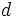$d$ satisfies the Leibniz rule: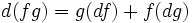$d(fg) = g(df) + f(dg)$

In other words,$d$ is a derivation from the algebra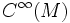$C^\infty(M)$ to the module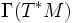$\Gamma(T^*M)$.

Proof

To verify equality of the two sides, we need to verify that for any vector field$X$, the two sides evaluate to the same thing for$X$. The left side, evaluated on$X$, yields: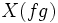$X(fg)$

The right side, evaluated on$X$, yields: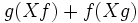$g(Xf) + f(Xg)$

The equality of these two sides follows from the Leibniz rule for derivations.